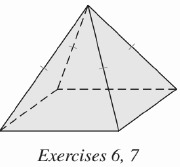Chapter 9.CT, Problem 6CT### Elementary Geometry for College St...

6th Edition
Daniel C. Alexander + 1 other
ISBN: 9781285195698

#### Solutions

Chapter
Section### Elementary Geometry for College St...

6th Edition
Daniel C. Alexander + 1 other
ISBN: 9781285195698
Textbook Problem
1 views

# Find the height of the regular square pyramid shown if each edge of the base measures 8 in. and the length of the slant height is 5 in. ____________To determine

To find:

The height of the regular square pyramid.

Explanation

Given:

For a regular square pyramid with each edge of the base measures 8 in. and the length of the slant height is 5 in.

Formula used:

In a regular pyramid, l2=a2+h2 where a is the apothem and h is the height.

Calculation:

We know that the length of the apothem of the square is one half of the length of the edge.

Therefore a=82=4 in

We have l=5 in

Thus l2=a2+h2 becomes

### Still sussing out bartleby?

Check out a sample textbook solution.

See a sample solution

#### The Solution to Your Study Problems

Bartleby provides explanations to thousands of textbook problems written by our experts, many with advanced degrees!

Get Started

#### Find y and y. y=lnx1+lnx

Single Variable Calculus: Early Transcendentals, Volume I

#### In Exercises 3540, rationalize the numerator of each expression. 37. 133

Applied Calculus for the Managerial, Life, and Social Sciences: A Brief Approach

#### In Problems 13-26, graph the solution of each system of inequalities. 21.

Mathematical Applications for the Management, Life, and Social Sciences

#### Using for |x| < 1,

Study Guide for Stewart's Multivariable Calculus, 8th